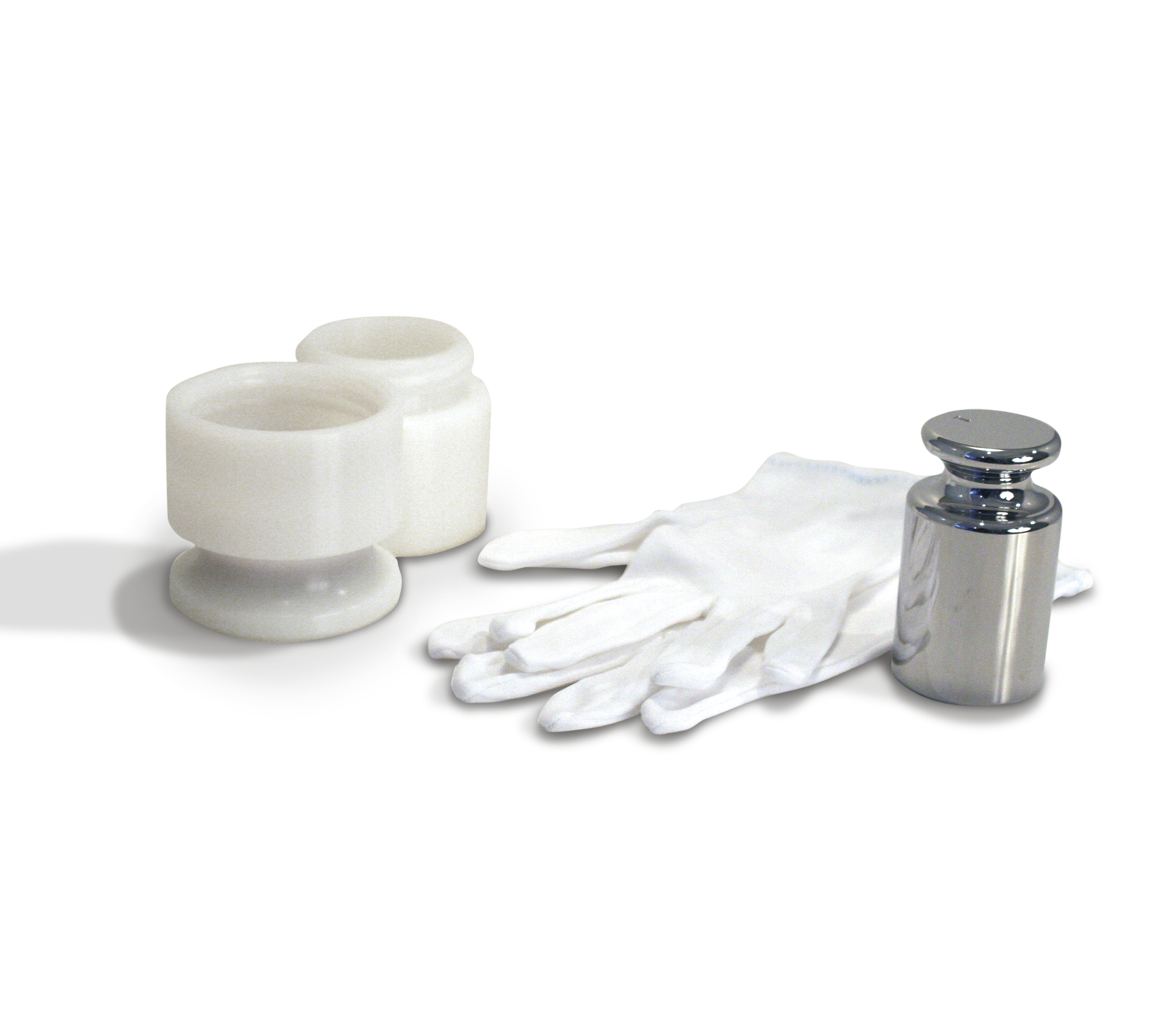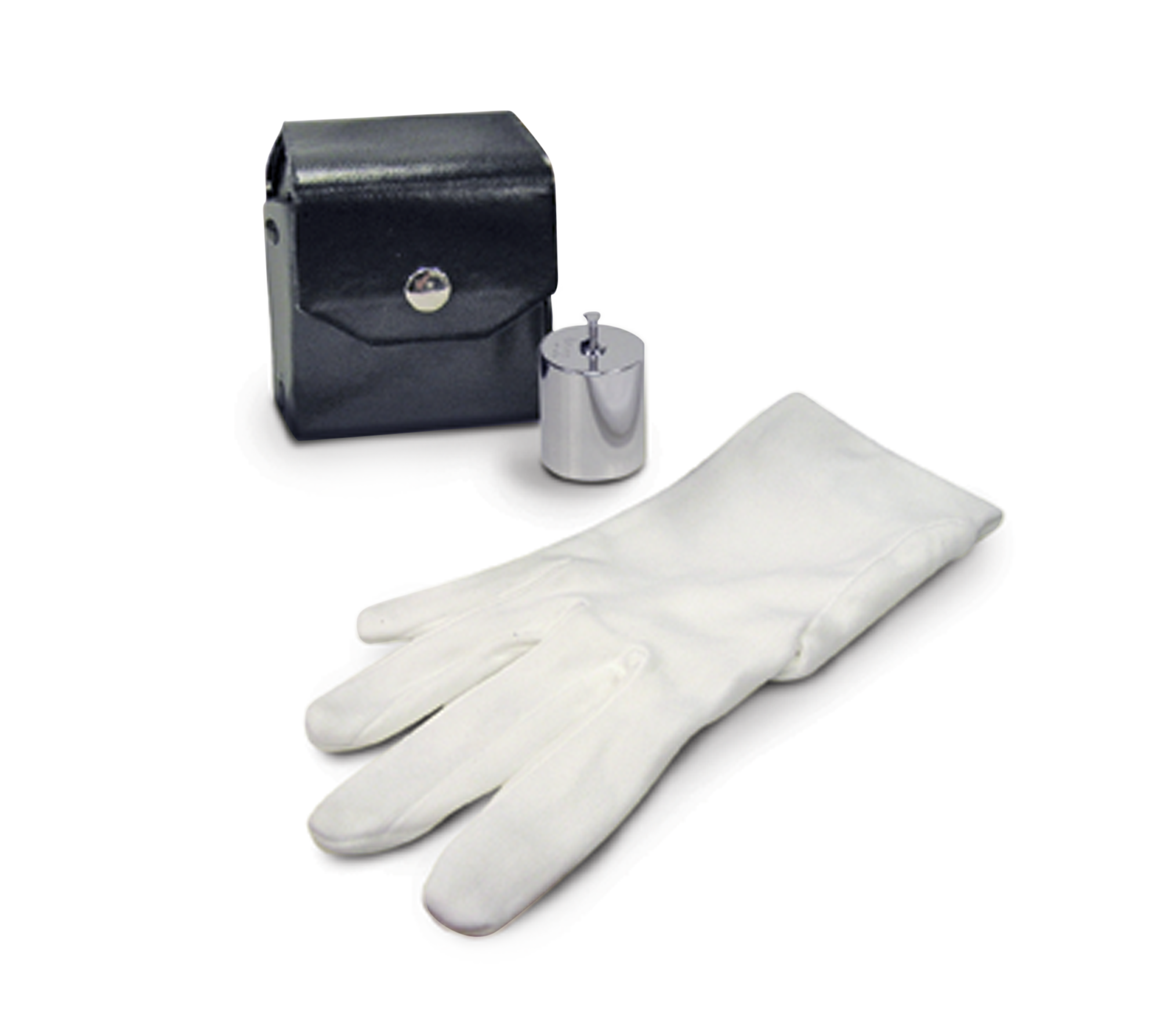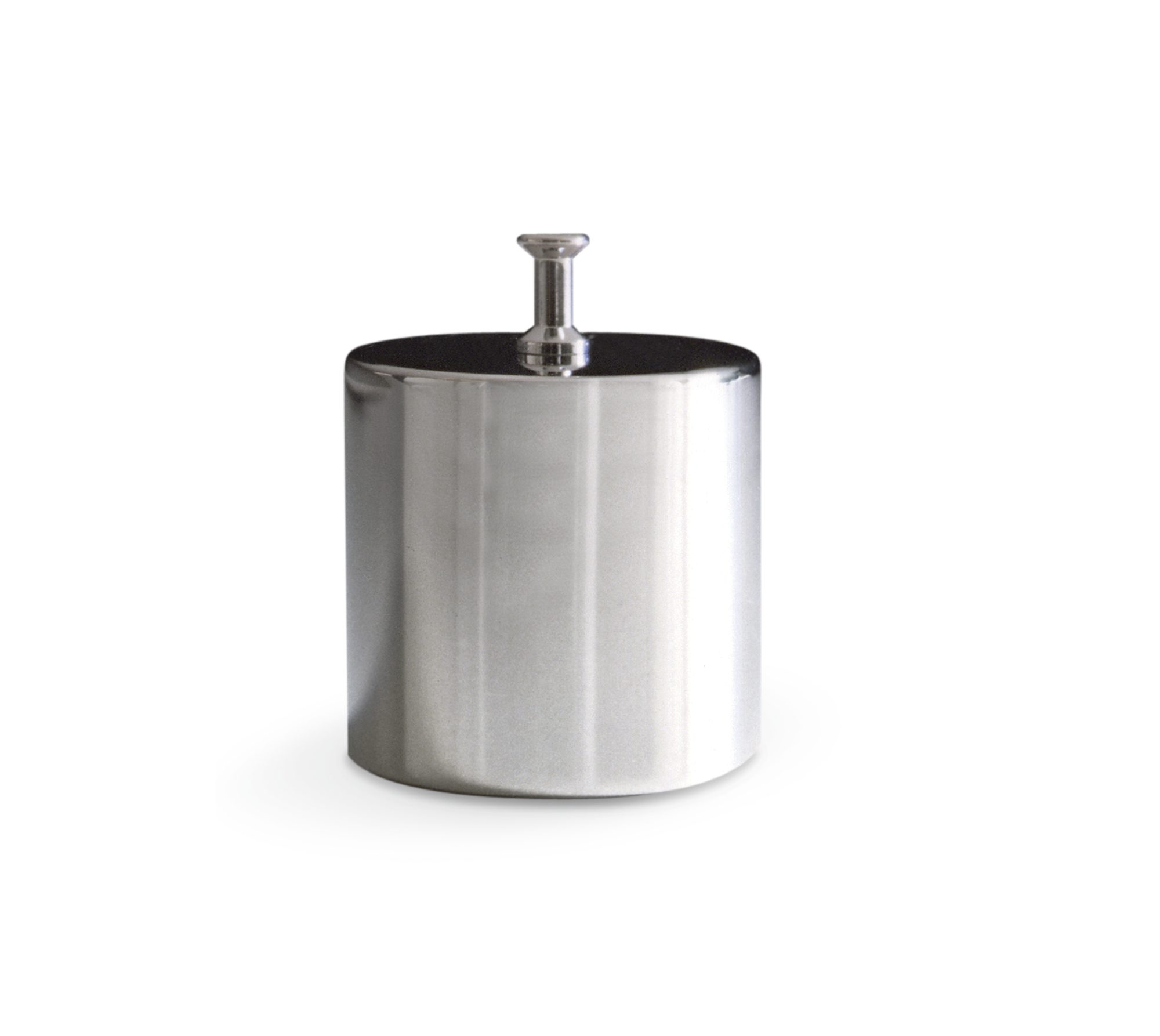Is the website displaying in the correct language? Please confirm or select a different language.

Your region has been set automatically. Please confirm or select a different region.

# ASTM Precision Laboratory Calibration Weights

Using ASTM precision calibration weights and mass reference standards helps ensure accuracy in laboratory weighing processes. Rice Lake offers ASTM calibration weights in Class 0, 1, 2, 3 and 4 as individual weights, sets and complete kits.## Single Calibration Weight 1 mg up to 25 kg Kit, ASTM Class 0

ASTM precision laboratory metric calibration kits are used when calibrating Class I and II balances, ASTM Class 4, 5 and 6 weights, student laboratory use, rough weighing operations, tolerance testing and verification checks.## Single Calibration Weight 0.05 mg up to 50 kg Kit, ASTM Class 1

ASTM precision laboratory metric calibration kits are used when calibrating Class I and II balances, ASTM Class 4, 5 and 6 weights, student laboratory use, rough weighing operations, tolerance testing and verification checks.## Single Calibration Weight 0.5 mg up to 50 kg Kit, ASTM Class 2

ASTM precision laboratory metric calibration kits are used when calibrating Class I and II balances, ASTM Class 4, 5 and 6 weights, student laboratory use, rough weighing operations, tolerance testing and verification checks.## Single Calibration Weight 0.05 mg up to 50 kg Kit, ASTM Class 3

ASTM precision laboratory metric calibration kits are used when calibrating Class I and II balances, ASTM Class 4, 5 and 6 weights, student laboratory use, rough weighing operations, tolerance testing and verification checks.## Single Calibration Weight 0.05 mg up to 50 kg Kit, ASTM Class 4

ASTM precision laboratory metric calibration kits are used when calibrating Class I and II balances, ASTM Class 4, 5 and 6 weights, student laboratory use, rough weighing operations, tolerance testing and verification checks.## Calibration Weight Kits, Precision Laboratory ASTM Class 1

ASTM precision laboratory avoirdupois kits are used when calibrating Class I and II balances, ASTM Class 4, 5 and 6 weights, student laboratory use, rough weighing operations, tolerance testing and verification checks.## Calibration Weight Kits, Precision Laboratory ASTM Class 2

ASTM precision laboratory avoirdupois kits are used when calibrating Class I and II balances, ASTM Class 4, 5 and 6 weights, student laboratory use, rough weighing operations, tolerance testing and verification checks.## Calibration Weight Kits, Precision Laboratory ASTM Class 3

ASTM precision laboratory avoirdupois kits are used when calibrating Class I and II balances, ASTM Class 4, 5 and 6 weights, student laboratory use, rough weighing operations, tolerance testing and verification checks.## ASTM Precision Laboratory Avoirdupois Calibration Weight Kits

ASTM precision laboratory avoirdupois kits are used when calibrating Class I and II balances, ASTM Class 4, 5 and 6 weights, student laboratory use, rough weighing operations, tolerance testing and verification checks.## Calibration Weights Avoirdupois Precision Laboratory, ASTM Class 1

ASTM precision laboratory avoirdupois individual weights are used when calibrating Class I and II balances, ASTM Class 4, 5 and 6 weights, student laboratory use, rough weighing operations, tolerance testing and verification checks.## Calibration Weights Avoirdupois Precision Laboratory, ASTM Class 2

ASTM precision laboratory avoirdupois individual weights are used when calibrating Class I and II balances, ASTM Class 4, 5 and 6 weights, student laboratory use, rough weighing operations, tolerance testing and verification checks.## Calibration Weights Avoirdupois Precision Laboratory, ASTM Class 3

ASTM precision laboratory avoirdupois individual weights are used when calibrating Class I and II balances, ASTM Class 4, 5 and 6 weights, student laboratory use, rough weighing operations, tolerance testing and verification checks.